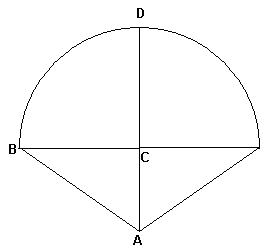From: Jordan How do you find the surface area of a cone with a hemisphere on top? The only information I have, is the radius of the hemisphere, which is 4, and the total height, which is 7. Hi Jordan. I've drawn a sketch of the middle cross-section of the shape so that we can label some points and dimensions on it.You know that the radius of the hemisphere, call this r, is 4 units and the total height is 7 units. Looking at the diagram, that means segment DC is 4 units (it is a radius of the hemisphere) and AC must be 3 units (7-4). Likewise, BC is a radius of the hemisphere, so it is 4 units also. If you know the radius of the base of a cone and the length of the slant, call it s, (that's segment AB in our diagram), then the surface area of the cone is πrs. But we don't yet have the length s. To figure out the length of the slant, you can use pythagorus, because triangle ABC is a right triangle and you know the lengths of the two legs. The surface area of a sphere is 4πr2, but a hemisphere would be just half of that. So when you add the surface areas together, you get the surface area of the whole shape. Hope this helps! Stephen La Rocque.